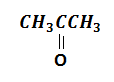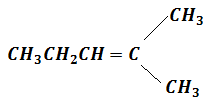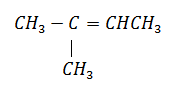In the reaction, ${\mathrm{C}}_{6}{\mathrm{H}}_{5}{\mathrm{CH}}_{3}\stackrel{\mathrm{Oxidation}}{\to }$$\stackrel{\mathrm{NaOH}}{\to }$B

the product C is:

1. ${\mathrm{C}}_{6}{\mathrm{H}}_{5}\mathrm{OH}$                              2. ${\mathrm{C}}_{6}{\mathrm{H}}_{6}$

3. ${\mathrm{C}}_{6}{\mathrm{H}}_{5}\mathrm{COONa}$                        4. ${\mathrm{C}}_{6}{\mathrm{H}}_{5}\mathrm{ONa}$

Concept Questions :-

Aromatic Hydrocarbons - Benzene - Structure, Preparation & Chemical Reactions
High Yielding Test Series + Question Bank - NEET 2020

Difficulty Level:

When acetylene is passed through dil. ${\mathrm{H}}_{2}{\mathrm{SO}}_{4}$ in presence of ${\mathrm{HgSO}}_{4}$, the compound formed is

1. ether                                    2. ketone

3. acetic acid                             4. acetaldehyde

Concept Questions :-

Alkanes, Alkenes and Alkynes - Chemical Properties
High Yielding Test Series + Question Bank - NEET 2020

Difficulty Level:

Acetylenic hydrogens are acidic because

1. sigma electron density of C-H bond in acetylene is nearer to carbon which has 50% s-character

2. acetylene has only one hydrogen on each carbon

3. acetylene contains least number of hydrogens among the possible hydrocarbons having two carbons

4. acetylene belong to the class of alkynes with molecular formula ${\mathrm{C}}_{\mathrm{n}}{\mathrm{H}}_{2\mathrm{n}-2}$

Concept Questions :-

Alkanes, Alkenes and Alkynes - Chemical Properties
High Yielding Test Series + Question Bank - NEET 2020

Difficulty Level:

A compound is treated with ${\mathrm{NaNH}}_{2}$ to give sodium salt. Identify the compound.

(a) ${\mathrm{C}}_{2}{\mathrm{H}}_{2}$                                 (b) ${\mathrm{C}}_{6}{\mathrm{H}}_{6}$

(c) ${\mathrm{C}}_{2}{\mathrm{H}}_{6}$                                 (d) ${\mathrm{C}}_{2}{\mathrm{H}}_{4}$

Concept Questions :-

Alkanes, Alkenes and Alkynes - Chemical Properties
High Yielding Test Series + Question Bank - NEET 2020

Difficulty Level:

Ethylene forms ethylene chlorohydrin by the action of:

1. dry chlorine gas

2. dry hydrogen chlorine gas

3. solution of chlorine gas in water

4. dilute hydrochloric acid

Concept Questions :-

Alkanes, Alkenes and Alkynes - Chemical Properties
High Yielding Test Series + Question Bank - NEET 2020

Difficulty Level:

Which alkene on ozonolysis gives ${\mathrm{CH}}_{3}{\mathrm{CH}}_{2}\mathrm{CHO}$ and?

(a)(b) ${\mathrm{CH}}_{3}{\mathrm{CH}}_{2}\mathrm{CH}={\mathrm{CHCH}}_{2}{\mathrm{CH}}_{3}$

(c) ${\mathrm{CH}}_{3}{\mathrm{CH}}_{2}\mathrm{CH}={\mathrm{CHCH}}_{3}$

(d)Concept Questions :-

Alkanes, Alkenes and Alkynes - Chemical Properties
High Yielding Test Series + Question Bank - NEET 2020

Difficulty Level:

Hydroxylation of propyne in the presence of ${\mathrm{HgSO}}_{4}/{\mathrm{H}}_{2}{\mathrm{SO}}_{4}$ is initiated by the attack of:

3. electrophile                               4. nucleophile

Concept Questions :-

Alkanes, Alkenes and Alkynes - Chemical Properties
High Yielding Test Series + Question Bank - NEET 2020

Difficulty Level:

Ozonolysis of buta-1,3-diene gives:

1. HCHO and glyoxal

2. ${\mathrm{CH}}_{3}\mathrm{CHO}$ and glyoxal

3. ${\mathrm{CO}}_{2}$ and glyoxal

4. HCHO + glyoxal + ${\mathrm{CH}}_{3}\mathrm{CHO}$

Concept Questions :-

Alkanes, Alkenes and Alkynes - Chemical Properties
High Yielding Test Series + Question Bank - NEET 2020

Difficulty Level:

A mixture of ethane, ethene and ethyne is passed through ammoniacal ${\mathrm{AgNO}}_{3}$ solution. The gases which remain unreacted are:

1. ethane and ethene                 2. ethane and ethyne

3. ethene and ethyne                 4. ethane only

Concept Questions :-

Alkanes, Alkenes and Alkynes - Chemical Properties
High Yielding Test Series + Question Bank - NEET 2020

Difficulty Level:

Which is the most suitable reagent among the following to distinguish compound (III) from rest of the compounds?

I. ${\mathrm{CH}}_{3}-\mathrm{C}\equiv \mathrm{C}-{\mathrm{CH}}_{3}$

II. ${\mathrm{CH}}_{3}-{\mathrm{CH}}_{2}-{\mathrm{CH}}_{2}-{\mathrm{CH}}_{3}$

III. ${\mathrm{CH}}_{3}-{\mathrm{CH}}_{2}-\mathrm{C}\equiv \mathrm{CH}$

IV. ${\mathrm{CH}}_{3}-\mathrm{CH}={\mathrm{CH}}_{2}$

1. ${\mathrm{Br}}_{2}$/${\mathrm{CCl}}_{4}$                                        2. ${\mathrm{Br}}_{2}$/${\mathrm{CH}}_{3}\mathrm{COOH}$

3. Alk.${\mathrm{KMnO}}_{4}$                                     4. Ammoniacal ${\mathrm{AgNO}}_{3}$

Concept Questions :-

Alkanes, Alkenes and Alkynes - Chemical Properties